# Direction Cosines and Direction Ratios of a Line JEE Notes | EduRev

## JEE : Direction Cosines and Direction Ratios of a Line JEE Notes | EduRev

The document Direction Cosines and Direction Ratios of a Line JEE Notes | EduRev is a part of the JEE Course Mathematics (Maths) Class 12.
All you need of JEE at this link: JEE

Direction Cosines Of A Line

Ifare the angles which a given directed line makes with the positive directions of the axes. of x, y and z respectively, then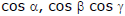are called the direction cosines (briefly written as d.c.’s) of the line. These d.c.’s are usually denote by l, m, n.
Let AB be a given line. Draw a line OP parallel to the line AB and passing through the origin O. Measure angles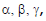thenare the d.c.’s of the line AB. It can be easily seen that l, m, n, are the direction cosines of a line if and only if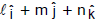is a unit vector in the direction of that line. Clearly OP'(i.e. the line through O and parallel to BA) makes angle 180° – α, 180°– β, 180° – γ with OX, OY and OZ respectively. Hence d.c.’s of the line BA are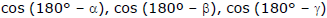i.e., are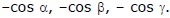If the length of a line OP through the origin O be r, then the co-ordinates of P are (lr, mr, nr) where l, m, n are the d c.’s of OP.

If l, m, n are direction cosines of any line AB, then they will satisfy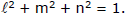Direction Ratios :

If the direction cosines l, m, n of a given line be proportional to any three numbers a, b, c respectively, then the numbers a, b, c are called direction ratios (briefly written as d.r.’s of the given line.

Relation Between Direction Cosines And Direction Ratios :

Let a, b, c be the direction ratios of a line whose d.c.’s are l, m, n. From the definition of d.r.’s. we have l/a = m/b = n/c = k (say).     Then l = ka, m = kb, n = kc. But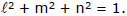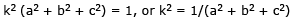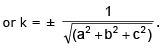.
Taking the positive value of k, we get l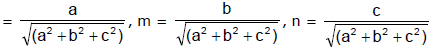Again taking the negative value of k, we get l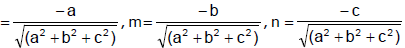Remark. Direction cosines of a line are unique. But the direction ratios of a line are by no means unique. If a, b, c are direction ratios of a line, then ka, kb, kc are also direction ratios of that line where k is any non-zero real number. Moreover if a, b, c are direction ratios of a line, then aiˆ + bjˆ + ckˆ is a vector parallel to that line.

Ex.4 Find the direction cosines l + m + n of the two lines which are connected by the relation l + m + n = 0 and mn – 2nl –2lm = 0.

Sol. The given relations are l + m + n = 0 or l = –m – n ....(1)

and mn – 2nl – 2lm = 0 ...(2)
Putting the value of l from (1) in the relation (2), we get
mn – 2n (–m –n) – 2(–m – n) m = 0 or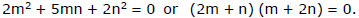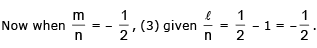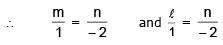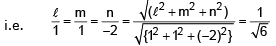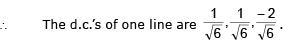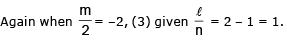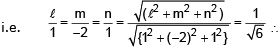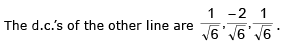To find the projection of the line joining two points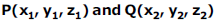on the another line whose d.c.’s are l, m, n.

Let O be the origin. Then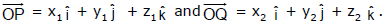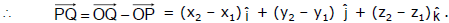Now the unit vector along the line whose d.c.’s are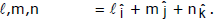∴ projection of PQ on the line whose d.c.’s are l, m, n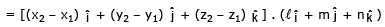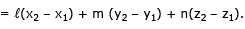The angle θ between these two lines is given by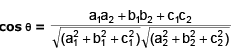If l1, m1, n1 and l2 , m2, n2 are two sets of real numbers, then

(l12 + m12 + n12) (l22 + m22 + n22) - (l1l2 + m1m2 + n1n2)2 = (m1n2 – m2n1)2 + (n1l2 - n2l1)2 + (l1m2 - l2m1)2

Now, we have

sin2θ = 1 - cos2θ = 1 - (l1l2 + m1m2 + n1n2)2 = (l12 + m12 + n12) (l22 + m22 + n22) - (l1l2 + m1m2 + n1n2)2

= (m1n2 – m2n1)2 + (n1l2 - n2l1)2 + (l1m2 - l2m1)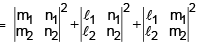Condition for perpendicularity ⇒ l1l2 + m1m2 + n1n2 = 0.

Condition for parallelism ⇒  l1 = l2, m1 = m2, n1 = n2. ⇒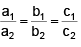Ex.5 Show that the lines whose d.c.’s are given by l + m + n = 0 and 2mn + 3ln - 5lm = 0 are at right angles.

Sol. From the first relation, we have l = -m - n....(1)

Putting this value of l in the second relation, we have

2mn + 3 (–m –n) n – 5 (–m –n) m = 0 or 5m2 + 4mn – 3n2 = 0 or 5(m/n)2 + 4(m/n) – 3 = 0 ....(2)

Let l1, m1, n1 and l2, m2, n2 be the d,c,s of the two lines. Then the roots of (2) are m1/n1 and m2/n2.

product of the roots =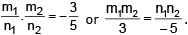...(3)

Again from (1), n = – l - m and putting this value of n in the second given relation, we have

2m (–l - m) + 3l(-l - m) - 5lm = 0  or 3(l/m)2 + 10 (l/m) + 2 = 0.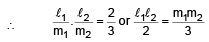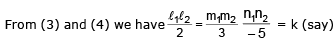l1l2 + m1m2 + n1n= (2 + 3 - 5) k = 0 . k = 0.  ⇒  The lines are at right angles.

Remarks :

(a) Any three numbers a, b, c proportional to the direction cosines are called the direction ratios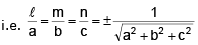same sign either +ve or –ve should be taken throughout.

Note that d.r’s of a line joining x1, y1, z1 and x2, y2, z2 are proportional to x2 – x1, y2 – y1 and z2 – z1

(b) If θ is the angle between the two lines whose d.c's are l 1 , m1, n1 and l 2 , m2, n2

cos θ = l1l2 + m1m2 + n1n2

Hence if lines are perpendicular then l1l2 + m1m2 + n1n2 = 0.

if lines are parallel then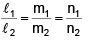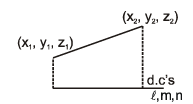Note that if three lines are coplanar then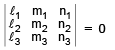(c) Projection of the join of two points on a line with d.c’s l, m, n are l (x2 – x1) + m(y2 – y1) + n(z2 – z1)

(d) If l1, m1, n1 and l2, m2, n2 are the d.c.'s of two concurrent lines, show that the d.c's of two lines bisecting the angles between them are proportional to l1 ± l2, m1 ± m2, n1 ± n2

Offer running on EduRev: Apply code STAYHOME200 to get INR 200 off on our premium plan EduRev Infinity!

## Mathematics (Maths) Class 12

209 videos|222 docs|124 tests

,

,

,

,

,

,

,

,

,

,

,

,

,

,

,

,

,

,

,

,

,

;